# reflectorSpherical

Create spherical reflector-backed antenna

Since R2020b

## Description

The `reflectorSpherical` antenna object creates a spherical reflector-backed antenna. The reflector in the spherical reflector-backed antenna is one-half the size of the sphere. The antenna is used in wide-angle scanning on account of its perfectly symmetrical geometric configuration.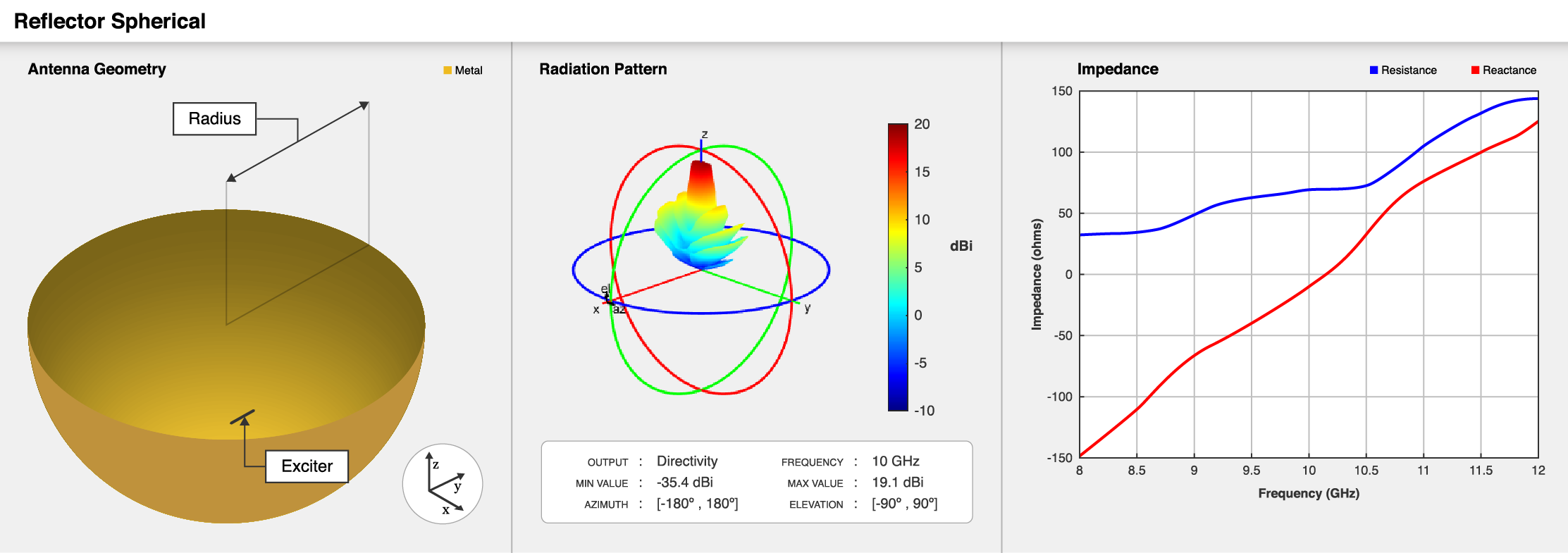## Creation

### Syntax

``ant = reflectorSpherical``
``ant = reflectorSpherical(Name=Value)``

### Description

example

````ant = reflectorSpherical` creates a spherical reflector-backed antenna. The default antenna object has an exciter as a center-fed dipole located on the X-Y plane. The default antenna object dimensions are chosen for an operating frequency of 1 GHz.```

example

````ant = reflectorSpherical(Name=Value)` creates a cavity-backed antenna, with additional Properties specified by one or more name–value arguments. `Name` is the property name and `Value` is the corresponding value. You can specify several name-value arguments in any order as `Name1`= `Value1`, `...`, `NameN`=`ValueN`. Properties not specified retain their default values.For example, `reflectorSpherical(Radius=0.6)` sets the spherical reflector radius to 0.6 meters.```

## Properties

expand all

Exciter antenna or array type, specified as an antenna object, an array object, measured pattern data of an antenna, or an empty array. Except for reflector and cavity antenna elements, you can use any Antenna Toolbox™ antenna or array element as an exciter. To create the reflector backing structure without the exciter, specify this property as an empty array.

Example: `dipole`

Example: `linearArray(Element=patchMicrostrip)`

Example: `measuredAntenna`

Example: `[]`

Aperture radius of the spherical reflector along X and Y-axes, specified as a positive scalar in meters.

Example: `0.259`

Data Types: `double`

Signed distance between feed point of the exciter and the origin, specified as a three-element vector with each element unit in meters.

Example: `[0 0 0.082]`

Data Types: `double`

Perpendicular distance between origin and the aperture of the spherical reflector-backed antenna, specified as a positive scalar in meters.

Example: `0.6`

Note

`Depth` should be less than or half the Radius.

Data Types: `double`

Type of the metal used as a conductor, specified as a metal material object. You can choose any metal from the `MetalCatalog` or specify a metal of your choice. For more information, see `metal`. For more information on metal conductor meshing, see Meshing.

Example: `metal("Copper")`

Tilt angle of the antenna in degrees, specified as a scalar or vector. For more information, see Rotate Antennas and Arrays.

Example: `90`

Example: `Tilt=[90 90]`,`TiltAxis=[0 1 0;0 1 1]` tilts the antenna at 90 degrees about the two axes defined by the vectors.

Data Types: `double`

Tilt axis of the antenna, specified as one of these values:

• Three-element vector of Cartesian coordinates in meters. In this case, each coordinate in the vector starts at the origin and lies along the specified points on the x-, y-, and z-axes.

• Two points in space, specified as a 2-by-3 matrix corresponding to two three-element vectors of Cartesian coordinates. In this case, the antenna rotates around the line joining the two points.

• `"x"`, `"y"`, or `"z"` to describe a rotation about the x-, y-, or z-axis, respectively.

Example: `[0 1 0]`

Example: `[0 0 0;0 1 0]`

Example: `"Z"`

Data Types: `double` | `string`

Lumped elements added to the antenna feed, specified as a `lumpedElement` object. You can add a load anywhere on the surface of the antenna. By default, the load is at the feed. For more information, see `lumpedElement`.

Example: `Load=lumpedelements`, where `lumpedelements` is the load added to the antenna feed.

Example: `lumpedElement(Impedance=75)`

Solver for antenna analysis, specified as a string. Default solver is `"MoM-PO"`(Method of Moments-Physical Optics hybrid). Other supported solvers are: `"MoM"` (Method of Moments), `"PO"` (Physical optics) or `"FMM"` (Fast Multipole Method).

Example: `SolverType`=`"MoM"`

Data Types: `string`

## Object Functions

 `show` Display antenna, array structures, shapes, or platform `solver` Access FMM solver for electromagnetic analysis `impedance` Input impedance of antenna or scan impedance of array `sparameters` Calculate S-parameters for antennas and antenna arrays `returnLoss` Return loss of antenna or scan return loss of array `vswr` Voltage standing wave ratio (VSWR) of antenna or array element `optimize` Optimize antenna or array using SADEA optimizer `pattern` Plot radiation pattern and phase of antenna or array or embedded pattern of antenna element in array `patternAzimuth` Azimuth plane radiation pattern of antenna or array `patternElevation` Elevation plane radiation pattern of antenna or array `axialRatio` Axial ratio of antenna `beamwidth` Beamwidth of antenna `current` Current distribution on antenna or array surface `charge` Charge distribution on antenna or array surface `efficiency` Radiation efficiency of antenna `EHfields` Electric and magnetic fields of antennas or embedded electric and magnetic fields of antenna element in arrays `mesh` Mesh properties of metal, dielectric antenna, or array structure `design` Design prototype antenna or arrays for resonance around specified frequency or create AI-based antenna from antenna catalog objects `rcs` Calculate and plot radar cross section (RCS) of platform, antenna, or array

## Examples

collapse all

Create a spherical reflector-backed antenna object with default properties.

`ant = reflectorSpherical`
```ant = reflectorSpherical with properties: Exciter: [1x1 dipole] Radius: 0.1500 Depth: 0.1500 FeedOffset: [0 0 0.0750] Tilt: 0 TiltAxis: [1 0 0] Load: [1x1 lumpedElement] SolverType: 'MoM-PO' ```

View the antenna.

`show(ant)`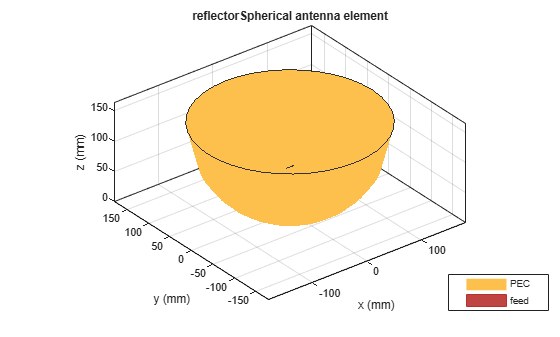Create a spherical reflector-backed antenna with a dipole as an exciter spaced at 90 millimeters.

```rs = reflectorSpherical; rs.FeedOffset(3) = 90e-3;```

Visualize the antenna.

```figure show(rs)```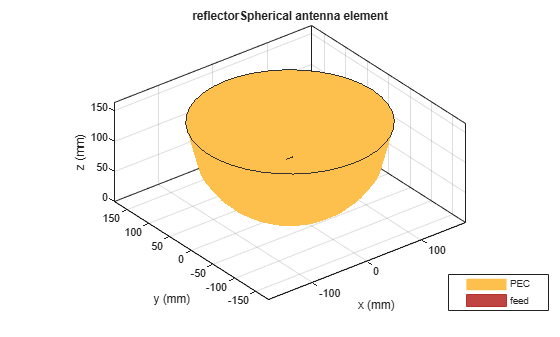Plot the S-parameters at 1 GHz.

```s = sparameters(rs,(9:0.1:11)*1e9); figure rfplot(s)```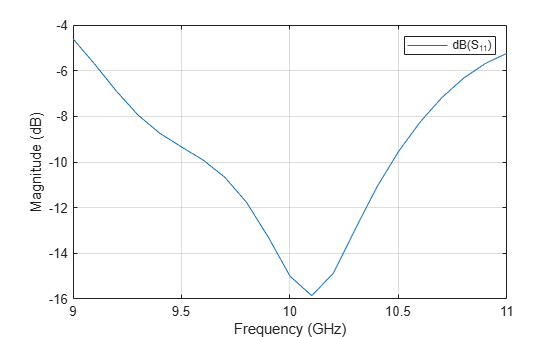Create a waveguide designed at 10 GHz backed with a spherical reflector.

```w = design(waveguide,10e9); rs = reflectorSpherical(Exciter=w); rs.Exciter.Tilt = 90; rs.Exciter.TiltAxis = [ 0 1 0];```

Visualize the antenna.

```figure show(rs)```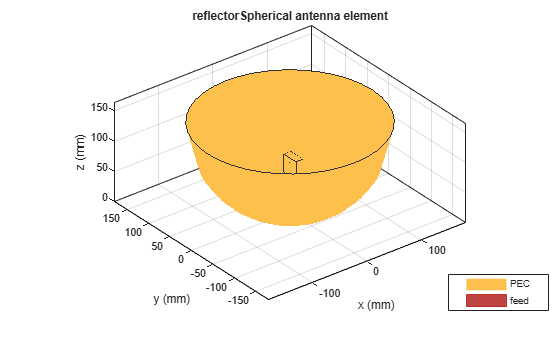Plot the radiation pattern at 10 GHz.

```figure pattern(rs,10e9)```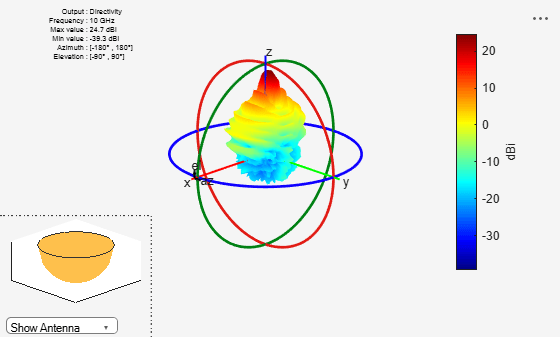Create a circular array with discone antennas.

```d = discone(Height=0.04); circArr = circularArray(Element=d,Radius=0.1);```

Create a spherical reflector antenna with circular array exciter.

`ant = reflectorSpherical(Exciter=circArr,Radius=0.25)`
```ant = reflectorSpherical with properties: Exciter: [1x1 circularArray] Radius: 0.2500 Depth: 0.1500 FeedOffset: [0 0 0.0750] Tilt: 0 TiltAxis: [1 0 0] Load: [1x1 lumpedElement] SolverType: 'MoM-PO' ```
`show(ant)`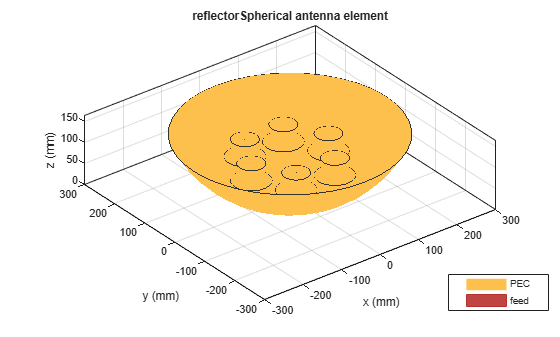Balanis, Constantine A. Antenna Theory: Analysis and Design. 3rd ed. Hoboken, NJ: John Wiley, 2005.